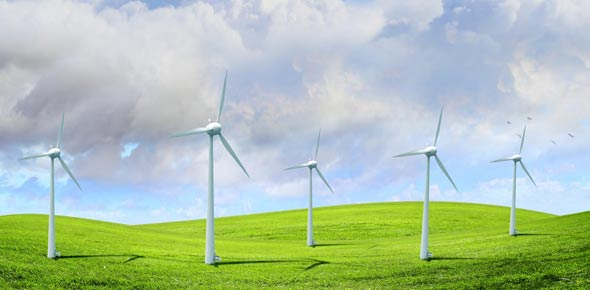# Renewable Sources Of Energy

13 Questions | Total Attempts: 341SettingsRenewable Sources of Energy

• 1.
Energy cannot be created or destroyed. but can be changed from one form to another.
• A.

True

• B.

False

• 2.
________________ converts the energy of falling water into electricity.
• A.

Hydroelectric plants

• B.

Wind

• C.

Biomass

• 3.
________________ ferment farm waste and unwanted plants to produce methane gas.
• A.

Hydroelectric plants

• B.

Wind

• C.

Biomass

• 4.
________________converts energy of steam that comes from hot rock layers into electricity.
• A.

Hydroelectric plants

• B.

Wind

• C.

Biomass

• D.

Geothermal

• 5.
A force is push or pull that can cause an object to move, change its speed and direction and change its shape or size.
• A.

True

• B.

False

• 6.
An unbalanced force can change the motion of an object.
• A.

True

• B.

False

• 7.
_____________ is the tendency of an object to resist any change in its state of motion.
• A.

Unbalanced force

• B.

Enertia

• 8.
_____________ are forces in opposite directions and equal in size.
• A.

Balanced forces

• B.

Enertia

• 9.
Acceleration increases as force applied on an object increases.
• A.

True

• B.

False

• 10.
Acceleration decreases as the mass of the object decreases.
• A.

True

• B.

False

• 11.
The object at rest will remain at rest and an object in motion will continue to be in constant motion unless an external force acts on it.
• A.

True

• B.

False

• 12.
Boys of the same weight are pushing a box against each other.
• A.

Balanced

• B.

Unbalanced

• C.

Enertia

• 13.
If force is zero.
• A.

Balanced

• B.

Unbalanced

• C.

Enertia

Related TopicsBack to top